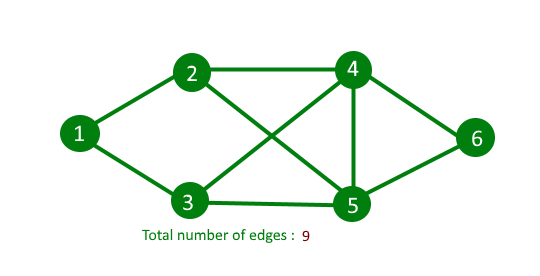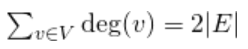# Count number of edges in an undirected graph

• Difficulty Level : Easy
• Last Updated : 10 Mar, 2022

Given an adjacency list representation undirected graph. Write a function to count the number of edges in the undirected graph.

Expected time complexity : O(V)

Examples:

```Input : Adjacency list representation of
below graph.
Output : 9```

## Recommended: Please try your approach on {IDE} first, before moving on to the solution.

Idea is based on Handshaking Lemma. Handshaking lemma is about undirected graph. In every finite undirected graph number of vertices with odd degree is always even. The handshaking lemma is a consequence of the degree sum formula (also sometimes called the handshaking lemma)

``

So we traverse all vertices, compute sum of sizes of their adjacency lists, and finally returns sum/2. Below implementation of above idea

## C++

 `// C++ program to count number of edge in``// undirected graph``#include``using` `namespace` `std;`` ` `// Adjacency list representation of graph``class` `Graph``{``    ``int` `V ;``    ``list < ``int` `> *adj;``public` `:``    ``Graph( ``int` `V )``    ``{``        ``this``->V = V ;``        ``adj = ``new` `list<``int``>[V];``    ``}``    ``void` `addEdge ( ``int` `u, ``int` `v ) ;``    ``int` `countEdges () ;``};`` ` `// add edge to graph``void` `Graph :: addEdge ( ``int` `u, ``int` `v )``{``    ``adj[u].push_back(v);``    ``adj[v].push_back(u);``}`` ` `// Returns count of edge in undirected graph``int` `Graph :: countEdges()``{``    ``int` `sum = 0;`` ` `    ``//traverse all vertex``    ``for` `(``int` `i = 0 ; i < V ; i++)`` ` `        ``// add all edge that are linked to the``        ``// current vertex``        ``sum += adj[i].size();`` ` ` ` `    ``// The count of edge is always even because in``    ``// undirected graph every edge is connected``    ``// twice between two vertices``    ``return` `sum/2;``}`` ` `// driver program to check above function``int` `main()``{``    ``int` `V = 9 ;``    ``Graph g(V);`` ` `    ``// making above shown graph``    ``g.addEdge(0, 1 );``    ``g.addEdge(0, 7 );``    ``g.addEdge(1, 2 );``    ``g.addEdge(1, 7 );``    ``g.addEdge(2, 3 );``    ``g.addEdge(2, 8 );``    ``g.addEdge(2, 5 );``    ``g.addEdge(3, 4 );``    ``g.addEdge(3, 5 );``    ``g.addEdge(4, 5 );``    ``g.addEdge(5, 6 );``    ``g.addEdge(6, 7 );``    ``g.addEdge(6, 8 );``    ``g.addEdge(7, 8 );`` ` `    ``cout << g.countEdges() << endl;`` ` `    ``return` `0;``}`

## Java

 `// Java program to count number of edge in ``// undirected graph``import` `java.io.*;``import` `java.util.*;`` ` `// Adjacency list representation of graph``class` `Graph ``{``    ``int` `V;``    ``Vector[] adj;`` ` `    ``//@SuppressWarnings("unchecked")``    ``Graph(``int` `V)``    ``{``        ``this``.V = V;``        ``this``.adj = ``new` `Vector[V];`` ` `        ``for` `(``int` `i = ``0``; i < V; i++)``            ``adj[i] = ``new` `Vector();``    ``}`` ` `    ``// add edge to graph``    ``void` `addEdge(``int` `u, ``int` `v)``    ``{``        ``adj[u].add(v);``        ``adj[v].add(u);``    ``}`` ` `    ``// Returns count of edge in undirected graph``    ``int` `countEdges()``    ``{``        ``int` `sum = ``0``;`` ` `        ``// traverse all vertex``        ``for` `(``int` `i = ``0``; i < V; i++)`` ` `            ``// add all edge that are linked to the``            ``// current vertex``            ``sum += adj[i].size();`` ` `        ``// The count of edge is always even because in``        ``// undirected graph every edge is connected``        ``// twice between two vertices``        ``return` `sum / ``2``;``    ``}``}`` ` `class` `GFG ``{`` ` `    ``// Driver Code``    ``public` `static` `void` `main(String[] args) ``throws` `IOException ``    ``{``        ``int` `V = ``9``;``        ``Graph g = ``new` `Graph(V);`` ` `        ``// making above shown graph``        ``g.addEdge(``0``, ``1``);``        ``g.addEdge(``0``, ``7``);``        ``g.addEdge(``1``, ``2``);``        ``g.addEdge(``1``, ``7``);``        ``g.addEdge(``2``, ``3``);``        ``g.addEdge(``2``, ``8``);``        ``g.addEdge(``2``, ``5``);``        ``g.addEdge(``3``, ``4``);``        ``g.addEdge(``3``, ``5``);``        ``g.addEdge(``4``, ``5``);``        ``g.addEdge(``5``, ``6``);``        ``g.addEdge(``6``, ``7``);``        ``g.addEdge(``6``, ``8``);``        ``g.addEdge(``7``, ``8``);`` ` `        ``System.out.println(g.countEdges());``    ``}``}`` ` `// This code is contributed by``// sanjeev2552`

## Python3

 `# Python3 program to count number of ``# edge in undirected graph `` ` `# Adjacency list representation of graph ``class` `Graph:``    ``def` `__init__(``self``, V):``        ``self``.V ``=` `V ``        ``self``.adj ``=` `[[] ``for` `i ``in` `range``(V)]`` ` `    ``# add edge to graph ``    ``def` `addEdge (``self``, u, v ):``        ``self``.adj[u].append(v) ``        ``self``.adj[v].append(u)``     ` `    ``# Returns count of edge in undirected graph ``    ``def` `countEdges(``self``):``        ``Sum` `=` `0``     ` `        ``# traverse all vertex ``        ``for` `i ``in` `range``(``self``.V):``     ` `            ``# add all edge that are linked ``            ``# to the current vertex ``            ``Sum` `+``=` `len``(``self``.adj[i]) ``     ` `        ``# The count of edge is always even  ``        ``# because in undirected graph every edge  ``        ``# is connected twice between two vertices ``        ``return` `Sum` `/``/` `2`` ` `# Driver Code``if` `__name__ ``=``=` `'__main__'``:``     ` `    ``V ``=` `9``    ``g ``=` `Graph(V) `` ` `    ``# making above shown graph ``    ``g.addEdge(``0``, ``1` `) ``    ``g.addEdge(``0``, ``7` `) ``    ``g.addEdge(``1``, ``2` `) ``    ``g.addEdge(``1``, ``7` `) ``    ``g.addEdge(``2``, ``3` `) ``    ``g.addEdge(``2``, ``8` `) ``    ``g.addEdge(``2``, ``5` `) ``    ``g.addEdge(``3``, ``4` `) ``    ``g.addEdge(``3``, ``5` `) ``    ``g.addEdge(``4``, ``5` `) ``    ``g.addEdge(``5``, ``6` `) ``    ``g.addEdge(``6``, ``7` `) ``    ``g.addEdge(``6``, ``8` `) ``    ``g.addEdge(``7``, ``8` `) `` ` `    ``print``(g.countEdges())`` ` `# This code is contributed by PranchalK`

## C#

 `// C# program to count number of edge in ``// undirected graph``using` `System;``using` `System.Collections.Generic;`` ` `// Adjacency list representation of graph``class` `Graph ``{``    ``public` `int` `V;``    ``public` `List<``int``>[] adj;``    ``public` `Graph(``int` `V)``    ``{``        ``this``.V = V;``        ``this``.adj = ``new` `List<``int``>[V];`` ` `        ``for` `(``int` `i = 0; i < V; i++)``            ``adj[i] = ``new` `List<``int``>();``    ``}`` ` `    ``// add edge to graph``    ``public` `void` `addEdge(``int` `u, ``int` `v)``    ``{``        ``adj[u].Add(v);``        ``adj[v].Add(u);``    ``}`` ` `    ``// Returns count of edge in undirected graph``    ``public` `int` `countEdges()``    ``{``        ``int` `sum = 0;`` ` `        ``// traverse all vertex``        ``for` `(``int` `i = 0; i < V; i++)`` ` `            ``// add all edge that are linked to the``            ``// current vertex``            ``sum += adj[i].Count;`` ` `        ``// The count of edge is always even because in``        ``// undirected graph every edge is connected``        ``// twice between two vertices``        ``return` `sum / 2;``    ``}``}`` ` `class` `GFG ``{`` ` `    ``// Driver Code``    ``public` `static` `void` `Main(String[] args)``    ``{``        ``int` `V = 9;``        ``Graph g = ``new` `Graph(V);`` ` `        ``// making above shown graph``        ``g.addEdge(0, 1);``        ``g.addEdge(0, 7);``        ``g.addEdge(1, 2);``        ``g.addEdge(1, 7);``        ``g.addEdge(2, 3);``        ``g.addEdge(2, 8);``        ``g.addEdge(2, 5);``        ``g.addEdge(3, 4);``        ``g.addEdge(3, 5);``        ``g.addEdge(4, 5);``        ``g.addEdge(5, 6);``        ``g.addEdge(6, 7);``        ``g.addEdge(6, 8);``        ``g.addEdge(7, 8);`` ` `        ``Console.WriteLine(g.countEdges());``    ``}``}`` ` `// This code is contributed by PrinciRaj1992`

Output:
```14
```

Time Complexity: O(V)

This article is contributed by Nishant Singh. If you like GeeksforGeeks and would like to contribute, you can also write an article using write.geeksforgeeks.org or mail your article to review-team@geeksforgeeks.org. See your article appearing on the GeeksforGeeks main page and help other Geeks.